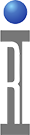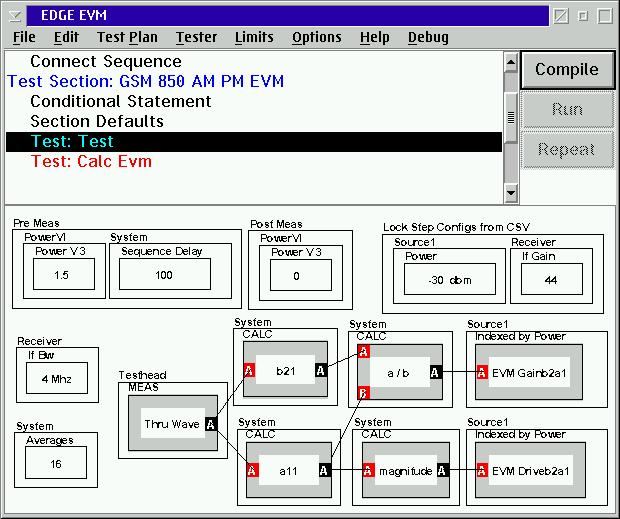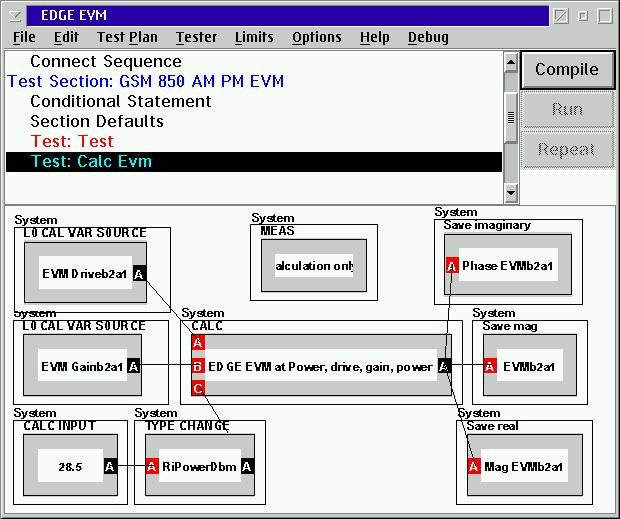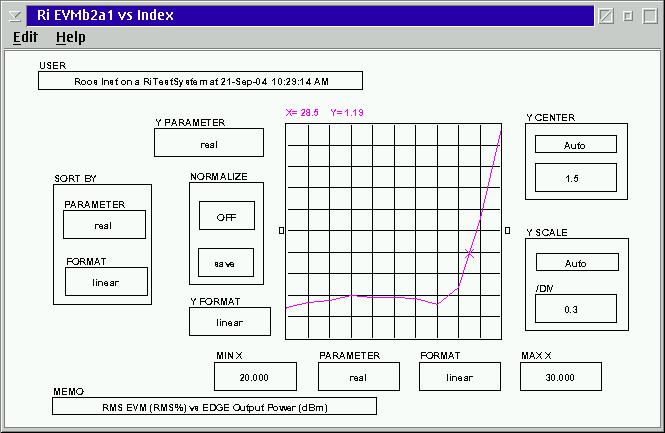Roos Instruments has developed a proprietary technique for EVM measurements that is not susceptible to many of the errors and time penalties of conventional EVM measurements. More information on the technique is available by contacting [email protected].

The basic premise is to characterize the DUT for all the amplitude and phase conditions it will see during the EDGE pulse constellation and then calculate the EVM for all the specific "chips" of an EDGE burst. To do this we have developed a calculation that takes the complex gain (in volts), the magnitude of the input wave (in volts) across the same range, and the Power out specified for the EVM value.

The following is an example of a large dynamic range EVM Measurement Panel:
Note:
1.) The actual measurement is a "Pick Meas" from a Test Head measure button. This "Thru Wave" measurement samples the input and output wave sequentially for maximum repeatability.
2.) An enhanced Lock Step function is used to allow multiple test conditions to be setup in a spreadsheet utilizing a CSV (comma separated variable) file. For info on usage see : Lock Step Configs from CSV.
3.) The device gain must be measured over a dynamic ranged from at least 14 dB below the desired EDGE Pout to at least 3dB above it. If the dynamic range of the test data is insufficient, the ideal EDGE modulation will not be able to be covered and the EVM calculation will not be able to be solved.Next is the EVM Calculation Panel:
Note:
1.) The desired Pout search value must be changed to the proper units by reflecting the User input data as the proper RI data type ( i.e RiPowerDbm )
2.) This is a Calc Only panel.
3.) The output of the calculation is a vector. We are using the vector data type to contain the Imaginary component as the RMS Phase EVM, Real component as RMS Mag EVM, and by utilizing a Magnitude calculation on the vector data, the composite RMS EVM.
4.) To report in any of these as a percent (%) multiply by 100.
5.) The calculation is for the data type RiComplexV.1.) There will be warnings during compile. Due to the nature of the compilation, which uses random values to check data flow, there will be some warnings (not errors) Real data will not have this problem, unless it is of insufficient range (see below)

2.) If the part is not measured over a sufficient dynamic range, (-14 dB to +3 dB relative to desired Pout) there will be warnings during a run.

3.) It is possible to range the "Power" input of the calculation (by ranging index and using an instrument state source of index) and display EVM vs Pout.

Physical considerations:

Since the b2 and a1 measurements are measured at the same tester conditions, care should be taken in the fixture design to keep the sampled waves as similar in amplitude as possible. This enables the optimum performance for the tester. For instance, if the DUT has a gain of 30dB, a 30 dB attenuator on the output would be appropriate.

Typical Edge EVM curve vs Output Power for an EDGE PA Module.https://roos.com/docs/MFEY-652MGE
ROOS INSTRUMENTS CONFIDENTIAL AND PROPRIETARY